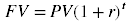×

# Future Value of Lump Sum Calculator

 Present value of lump sum : Interest rate per period: % Number of compounding periods:

## About Future Value of Lump Sum Calculator

The online Future Value of Lump Sum Calculator helps you calculate the future value of lump sum based on a fixed interest rate per period.

## Lump Sum

A lump sum is a complete payment consisting of a single sum of money, as opposed to a series of payments made over time (such as an annuity).

## Formula

The future value of lump sum calculation formula is as following:Where:
FV = future value of lump sum
PV = future value of lump sum
r = interest rate per period
t = number of compounding periods

Our website uses cookies to improve your user experience. If you continue browsing, we assume that you consent to our use of cookies. More information can be found in our privacy policy
Deals